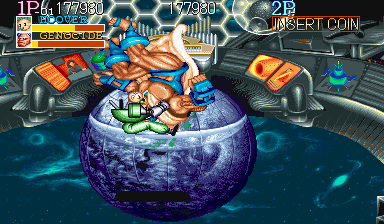## [街机名将][全敌将HP值精确测试][从小娃单挑羊头的最佳战法说起]

2009-04-20 16:32 | 火光

http://1lifeclear.ffsky.cn/35-4.htm

# 从小娃单挑羊头的最佳战法说起

[街机名将][Baby一命通关录像&攻略][日版双最高难度]

# 羊头的HP值精确测试4*2+12*4+4*2+6=70

12*13+2=158

X+5Y=158
X=12*3+n
1≤n≤4

Y=(158-X)/5
X=36+n
Y=(122-n)/5

# 火光对全敌将HP值的测算结果

## 第1关敌将：DOLG/看门狗

{}

24*2+72=48+72=120
120+20=140
120+16=136

## 第2关敌将：SHTROM.JY/黄狮/单狮

{}

24*3+72=72+72=144
144+4=148
144+7=151

## 第3关敌将：YAMATO/大和

{}

24*5+72=120+72=192192+9=201192+12=204所以，大和的HP值所在区间为(201,204]。

## 第4关敌将：MONSTER/三眼怪/猩猩

{}

24*4+72=96+72=168168+9=177168+12=180所以，三眼的HP值所在区间为(177,180]。

{}

## 第7关敌将：BLOOD/鬼脚七

{}

24*5+72=120+72=192192+9=201192+12=204所以，鬼脚七的HP值所在区间为(201,204]。

## 第8关敌将：DOPPEL/二重身/幻影/分身/复制人/克隆人

{}

24*5+72=120+72=192192+18=210192+20=212所以，复制人主体的HP值所在区间为(210,212]。

{}

15+24+72=39+72=111所以，复制人副体的HP值为111。

# 参考文献

[CPS1][名将][Captain Commando]伤害测试帖（作者：地道联盟）

# 相关文章

[街机名将][Mummy一命通关录像&攻略][日版双最高难度]

[街机名将][Captain一命通关录像&攻略][日版双最高难度]

[街机名将][忍者一命通关录像&攻略][日版双最高难度]

[街机名将][Baby一命通关录像&攻略][日版双最高难度]

--------------------------------